# Excel Formula auditing tools

Excel
When working with formulas in Excel, it is often necessary to find where a mistake was made. Excel provides very useful tools: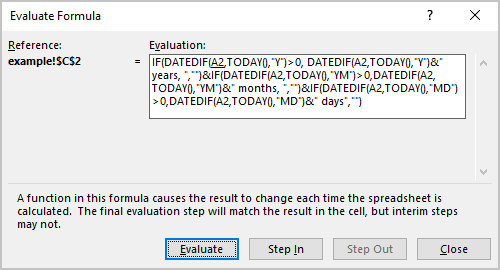Note: In Excel, formulas are evaluated in the natural order. In other words, if the formula in cell C2 depends on the result of the formula in cell A2, Excel evaluates cell A2 before calculating cell C2.

## A simple way to evaluate a part of a formula

You can use a simpler way to evaluate a part of a formula in Excel:

1.   Select the cell that contains the formula.

2.   Double-click the cell (or activate a cell and press F2) to get into Edit mode (see more about modifying cell data).

3.   Select the part of the formula you want to evaluate:

• Using the mouse:

Highlight the part of the formula: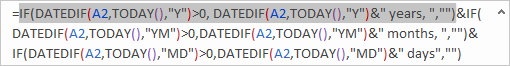• Using the keyboard:

Press Shift and use the navigation keys.

4.   Press F9.

Excel displays the calculated result of the selected part of the formula: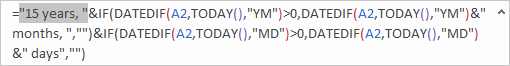5.   You can evaluate other parts of the formula: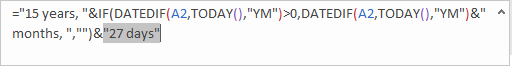or press Esc to cancel and return your formula to its previous state.

## Run the Formula Evaluator

The Evaluate Formula dialog box shows a formula being calculated one step at a time.

To run the Formula Evaluator, select the cell that contains the formula, and on the Formulas tab, in the Formula Auditing group, click Evaluate Formula: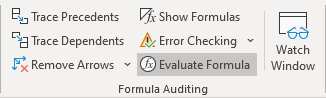In the Evaluate Formula dialog box:

• Click the Evaluate button repeatedly to see the intermediate results as the formula is calculated. The result of the evaluation is shown in italics: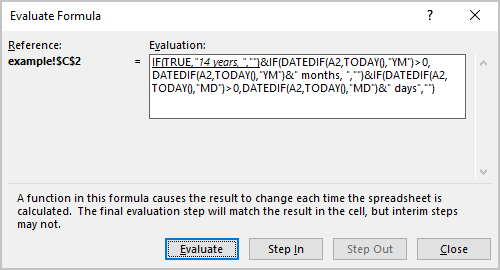• If the underlined part of the formula is a reference to another formula, click the Step In button to display the other formula in the Evaluation box: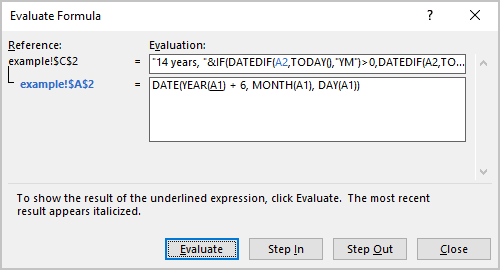• Click the Step Out button to go back to the previous cell and formula: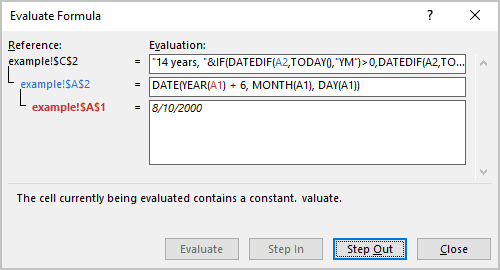• Click the Restart button to return the evaluation: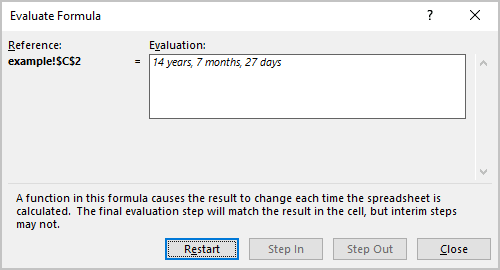## Tracing cell relationships

### Cell dependencies (in Microsoft Excel, cell precedents)

A formula cell dependencies are all the cells that contribute to the formula result. A direct dependency (in Microsoft Excel, direct precedents) is a cell you use directly in the formula. An indirect dependency (in Microsoft Excel, indirect precedents) is a cell that isn't used directly in the formula but is used by a cell you refer to.

For example: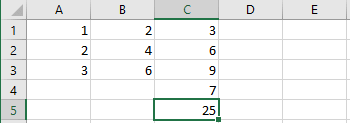• the cells C1:C3 contain the sum of the columns A and B,
• the cell C4 has a value of 7,
• the cell C5 contains the formula = SUM (C1:C4).

To see the cell dependencies, activate the cell, then on the Formulas tab, in the Formula Auditing group, click the Trace Precedents button: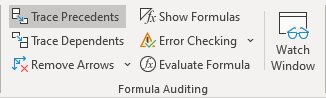Excel displays the direct dependencies for the cell C5:To see the next level of the dependencies, click the Trace Precedents button again: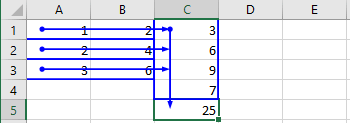Thus, to see all dependencies, you need to click the Trace Precedents button as many times as many precedent levels are used in the spreadsheet.

Notes:

1. If the sheet has a dependency on another sheet or workbook, you will see the arrow like this: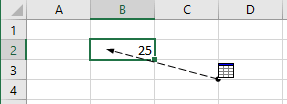### Cell dependents

Cell dependents include all formula cells that use the cell. The formula cell can be a direct dependent or an indirect dependent.

The example is the same as for the dependencies.

To see the cell dependents, activate the cell, then on the Formulas tab, in the Formula Auditing group, click the Trace Dependents button: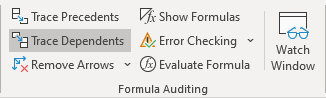Excel displays the direct dependents for the cell A1: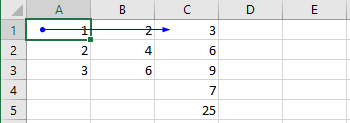To see the next level of the dependents, click the Trace Dependents button again: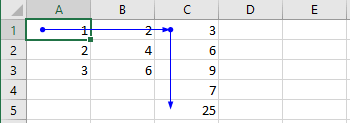Thus, to see all dependents, you need to click the Trace Dependents button as many times as many dependent levels are used in the spreadsheet.

Note: If the sheet has dependents in another sheet or even workbook, you will see the arrow like this: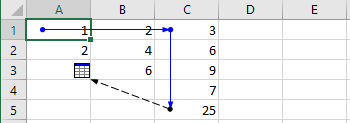## Remove arrows

To remove arrows, on the Formulas tab, in the Formula Auditing group:

• Click the Remove Arrows button to remove all arrows for precedents and dependents,
• Click the Remove Arrows button, then select Remove Precedent Arrows to remove only arrows for precedents,
• Click the Remove Arrows button, then select Remove Dependent Arrows to remove only arrows for dependents: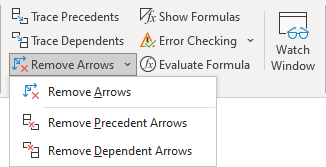See how to select special types of cells for more details.